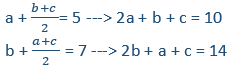# Let A, B and C be three positive integers such.. | Quantitative Aptitude - CAT Integers

## CAT 2020 - Slot 1 - Quantitative Aptitude - Integers - Question 8 - Let A, B and C be three positive integers such

Q. 8: Let A, B and C be three positive integers such that the sum of A and the mean of B and C is 5. In addition, the sum of B and the mean of A and C is 7. Then the sum of A and B is

##### 1.  62.  43.  74.  5Solving these two, we get b – a = 4

b = a + 4

Substituting this in the first equation

2a + a + 4 + c = 10

3a + c = 6

Given all three as positive integers, maximum value we can get for a = 1

Ex: When substituting a = 2, c = 0 (Not possible)

So a = 1, then b = 5

Sum of a + b = 6

### Past Year Question Paper & SolutionsCounselling Session
By IIM Mentor

#### Free Material Area